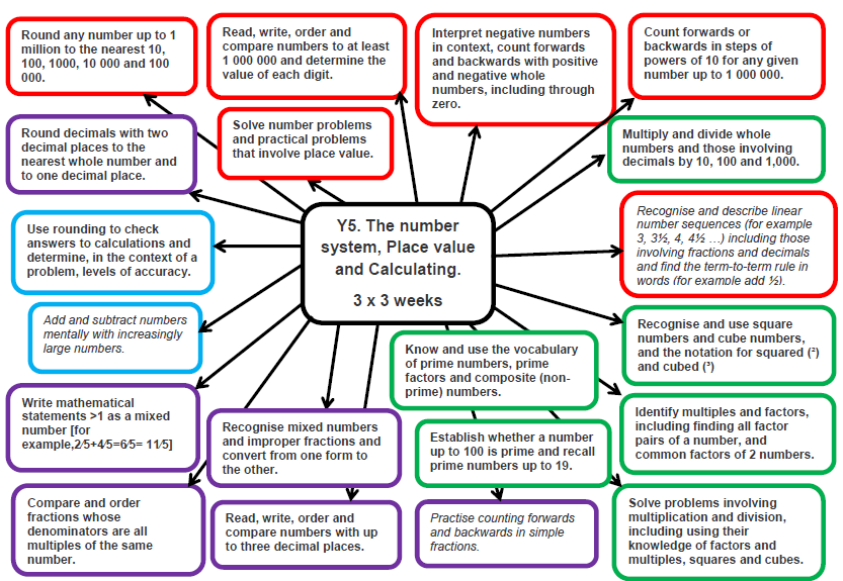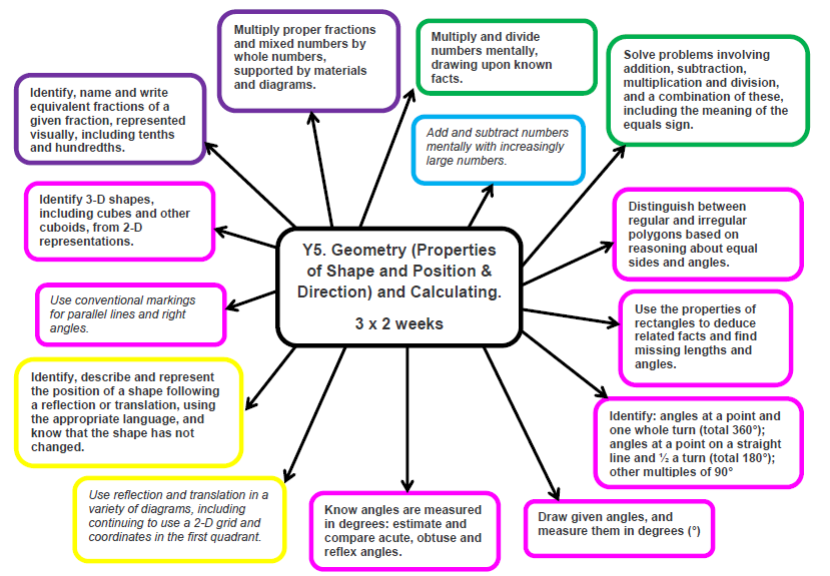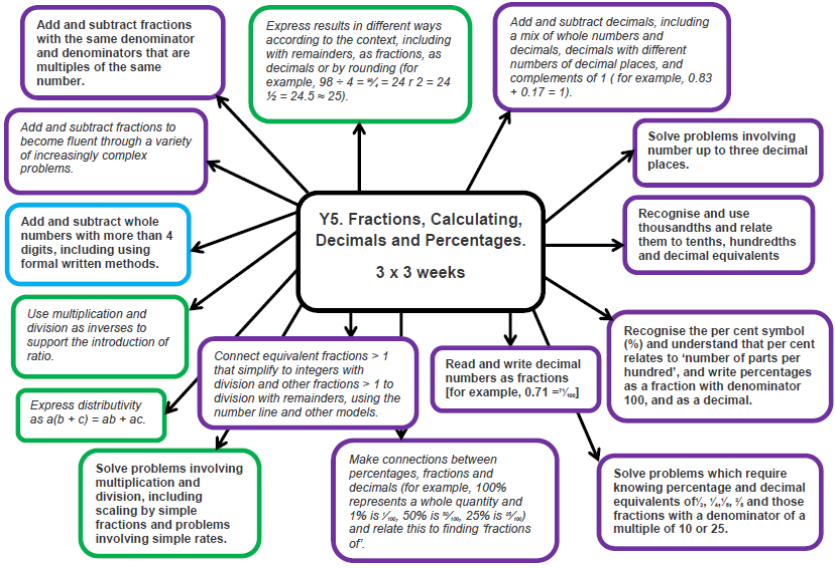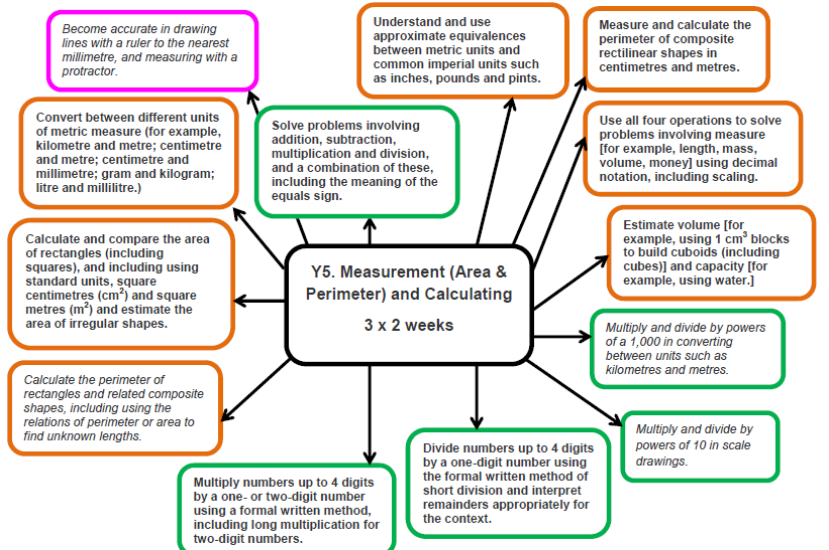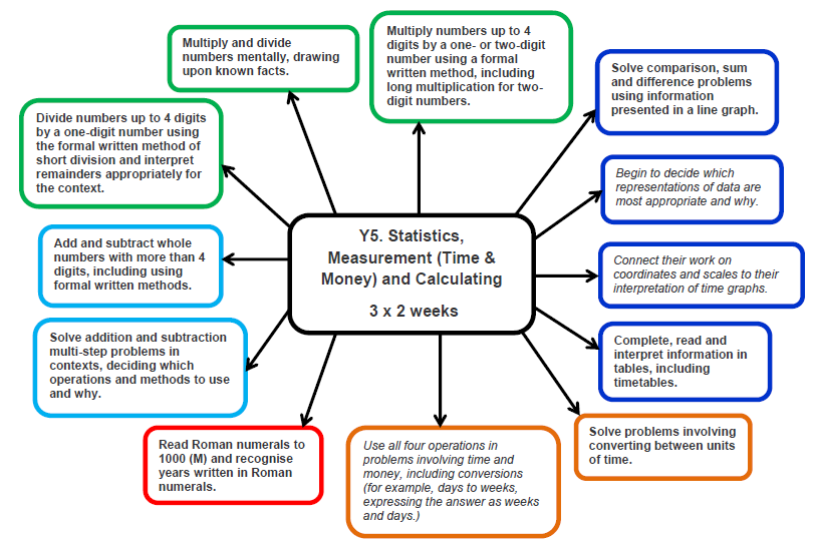## Developing Numeracy in Year 5

In Year 5 children work with numbers to at least 1,000,000. They will multiply and divide numbers up to 4 digits using formal written methods. They are taught to use negative numbers in context and understand prime numbers and prime factors. They will work confidently with decimal numbers and understand how fractions relate to decimal numbers and percentages. They will be taught to add and subtract fractions and recognise which fractions are equivalent. Children will be expected draw and measure angles to 360 degrees and will learn how to draw and reflect shapes. They will be taught to solve problems involving money and present information in a graphs, tables and charts.